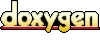# ACE_Stats_Value Class Reference

Helper class for ACE_Stats. More...

`#include <Stats.h>`

List of all members.

## Public Member Functions

ACE_Stats_Value (const u_int precision)
u_int precision (void) const
Accessor for precision.

void whole (const ACE_UINT32)
Set the whole_ field.

ACE_UINT32 whole (void) const
Accessor for the whole_ field.

void fractional (const ACE_UINT32)
Set the fractional_ field.

ACE_UINT32 fractional (void) const
Accessor for the fractional_ field.

ACE_UINT32 fractional_field (void) const
void scaled_value (ACE_UINT64 &) const
void dump (void) const
Print to stdout.

## Private Member Functions

ACE_Stats_Value (void)

## Private Attributes

ACE_UINT32 whole_
The integer portion of the value.

ACE_UINT32 fractional_
The fractional portion of the value.

u_int precision_

## Detailed Description

Helper class for ACE_Stats.

Container struct for 64-bit signed quantity and its precision. It would be nicer to use a fixed-point class, but this is sufficient. Users typically don't need to use this class directly; see ACE_Stats below.

Definition at line 41 of file Stats.h.

## Constructor & Destructor Documentation

 ACE_BEGIN_VERSIONED_NAMESPACE_DECL ACE_INLINE ACE_Stats_Value::ACE_Stats_Value ( const u_int precision )
 Constructor, which requires precision in terms of number of decimal digits. The more variation in the data, and the greater the data values, the smaller the precision must be to avoid overflow in the standard deviation calculation. 3 might be a good value, or maybe 4. 5 will probably be too large for non-trivial data sets. Definition at line 8 of file Stats.inl. ```00009 : whole_ (0), 00010 fractional_ (0), 00011 precision_ (precision) 00012 { 00013 } ```

 ACE_Stats_Value::ACE_Stats_Value ( void ) ` [inline, private]`
 Definition at line 85 of file Stats.h. ```00085 {} ```

## Member Function Documentation

 ACE_INLINE void ACE_Stats_Value::dump ( void ) const
 Print to stdout. Definition at line 59 of file Stats.inl. References ACE_DEBUG, ACE_LIB_TEXT, fractional_, LM_DEBUG, precision_, and whole_. ```00060 { 00061 #if defined (ACE_HAS_DUMP) 00062 ACE_DEBUG ((LM_DEBUG, 00063 ACE_LIB_TEXT ("precision: %u digits; whole: %u, fractional: %u\n"), 00064 precision_, whole_, fractional_)); 00065 #endif /* ACE_HAS_DUMP */ 00066 } ```

 ACE_INLINE ACE_UINT32 ACE_Stats_Value::fractional ( void ) const
 Accessor for the fractional_ field. Definition at line 45 of file Stats.inl. References fractional_. Referenced by scaled_value(). ```00046 { 00047 return fractional_; 00048 } ```

 ACE_INLINE void ACE_Stats_Value::fractional ( const ACE_UINT32 )
 Set the fractional_ field. Definition at line 38 of file Stats.inl. References fractional_. ```00039 { 00040 fractional_ = value; 00041 } ```

 ACE_BEGIN_VERSIONED_NAMESPACE_DECL ACE_UINT32 ACE_Stats_Value::fractional_field ( void ) const
 Calculates the maximum value of the fractional portion, given its precision. Definition at line 18 of file Stats.cpp. References precision(). Referenced by ACE_Stats::quotient(), scaled_value(), ACE_Stats::square_root(), and ACE_Stats::std_dev(). ```00019 { 00020 if (precision () == 0) 00021 { 00022 return 1; 00023 } 00024 else 00025 { 00026 ACE_UINT32 field = 10; 00027 for (u_int i = 0; i < precision () - 1; ++i) 00028 { 00029 field *= 10; 00030 } 00031 00032 return field; 00033 } 00034 } ```

 ACE_INLINE u_int ACE_Stats_Value::precision ( void ) const
 Accessor for precision. Definition at line 17 of file Stats.inl. References precision_. Referenced by fractional_field(), ACE_Stats::quotient(), ACE_Stats::square_root(), and ACE_Stats::std_dev(). ```00018 { 00019 return precision_; 00020 } ```

 ACE_INLINE void ACE_Stats_Value::scaled_value ( ACE_UINT64 & ) const
 Access the value as an _unsigned_ 64 bit quantity. It scales the value up by {precision} decimal digits, so that no precision will be lost. It assumes that {whole_} is >= 0. Definition at line 52 of file Stats.inl. References ACE_UINT64, fractional(), fractional_field(), and whole(). Referenced by ACE_Stats::std_dev(). ```00053 { 00054 sv = whole () * fractional_field () + fractional (); 00055 } ```

 ACE_INLINE ACE_UINT32 ACE_Stats_Value::whole ( void ) const
 Accessor for the whole_ field. Definition at line 31 of file Stats.inl. References whole_. Referenced by scaled_value(). ```00032 { 00033 return whole_; 00034 } ```

 ACE_INLINE void ACE_Stats_Value::whole ( const ACE_UINT32 )
 Set the whole_ field. Definition at line 24 of file Stats.inl. References whole_. ```00025 { 00026 whole_ = value; 00027 } ```

## Member Data Documentation

 ACE_UINT32 ACE_Stats_Value::fractional_` [private]`
 The fractional portion of the value. Definition at line 92 of file Stats.h. Referenced by dump(), and fractional().

 u_int ACE_Stats_Value::precision_` [private]`
 The number of decimal digits of precision represented by {fractional_}. Not declared const, so the only way to change it is via the assignment operator. Definition at line 99 of file Stats.h. Referenced by dump(), and precision().

 ACE_UINT32 ACE_Stats_Value::whole_` [private]`
 The integer portion of the value. Definition at line 89 of file Stats.h. Referenced by dump(), and whole().

The documentation for this class was generated from the following files:
Generated on Thu Nov 9 11:30:11 2006 for ACE by1.3.6